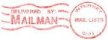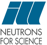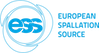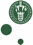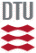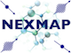McStas: Test_Sqw_monitor Instrument at Tests_samples

# The `Test_Sqw_monitor` Instrument

A simple ToF with cylindrical/spherical sample, and furnace/cryostat/container. The sample can be hollow.

## Identification

• Site: Tests_samples
• Author: E. Farhi
• Origin: ILL
• Date: Feb 2014
• Version:(Unknown)

## Description

```This instrument models a generic, tunable, neutron time-of-flight spectrometer.

The incoming flux at the sample brings neutrons with 'beam_wavelength_Angs' wavelength.
The energy resolution at the sample is given as 'beam_resolution_meV'.
The beam size at the sample is set to the sample cross section (e.g. ~2 x 5 cm).

The sample geometry is either cylindrical ('sample_radius_m' and 'sample_height_m')
or spherical ('sample_height_m=0'). The sample can be hollow when given a thickness
'sample_thickness_m', or filled ('sample_thickness_m=0'). When in cylindrical geometry,
it is surrounded by a container with thickness 'container_thickness_m'. The container
material is specified as a powder file name 'container' in the McStas Sqw or PowderN format,
e.g. 'Al.laz' or 'Nb.laz'. Setting 'container=0' removes the container.

The sample scattering is characterised by its coherent and incoherent contributions
given as 'sample_coh' and 'sample_inc' file names in McStas Sqw or PowderN format.
Setting the 'sample_coh=sample_inc=0' removes the sample, e.g. to study the container or
environment only contribution.

The sample and its container are located inside a cylindrical shield with radius
'environment_radius_m' and thickness 'environment_thickness_m'. The material is set from
the 'environment' file name in the McStas Sqw or PowderN format (e.g. 'Al.laz').
This way, it is possible to estimate the contribution of a cryostat or furnace.
Setting 'environment=0' removes the environment.

The detector has a cylindrical geometry, with a radius 'sample_detector_distance_m'
and tubes with height 'detector_height_m'. The detector covers a -30 to 140 deg angular range
with 2.54 cm diameter tubes (the number of tubes is computed from the distance).
The direct beam (non scattered neutrons) is discarded.

The detector produces both (angle,tof) and (q,w) data sets, for:
total scattering
coherent single scattering from sample
incoherent single scattering from sample
multiple scattering from sample
scattering from the container and sample environment
The (angle,tof) results are corrected for the parallax effect from the detector height.

Known configurations:
ILL_IN4:
beam_wavelength_Angs=2, beam_resolution_meV=0.5,
sample_detector_distance_m=2.0, detector_height_m=0.3
ILL_IN5:
beam_wavelength_Angs=5, beam_resolution_meV=0.1,
sample_detector_distance_m=4.0, detector_height_m=3.0
ILL_IN6:
beam_wavelength_Angs=4.1, beam_resolution_meV=0.1,
sample_detector_distance_m=2.48, detector_height_m=1.2
PSI_Focus:
beam_wavelength_Angs=4.1, beam_resolution_meV=0.1,
sample_detector_distance_m=2.5, detector_height_m=1.2
FRM2_TOFTOF:
beam_wavelength_Angs=3.0, beam_resolution_meV=0.3,
sample_detector_distance_m=4.0, detector_height_m=2.0
SNS_SEQUOIA:
beam_wavelength_Angs=1.0, beam_resolution_meV=3.0,
sample_detector_distance_m=5.5, detector_height_m=1.2
SNS_ARCS:
beam_wavelength_Angs=0.3, beam_resolution_meV=20.0,
sample_detector_distance_m=3.0, detector_height_m=1.46
NIST_DCS:
beam_wavelength_Angs=3.0, beam_resolution_meV=0.2,
sample_detector_distance_m=4.0, detector_height_m=1.2
ISIS_MERLIN:
beam_wavelength_Angs=1.0, beam_resolution_meV=2.4,
sample_detector_distance_m=2.5, detector_height_m=3.0
ISIS_LET:
beam_wavelength_Angs=4.0, beam_resolution_meV=0.1,
sample_detector_distance_m=3.5, detector_height_m=4.0

```

## Input parameters

Parameters in boldface are required; the others are optional.
Name Unit Description Default
beam_wavelength_Angs Angs incident neutron beam wavelength 2
beam_resolution_meV meV incident energy range full width 0.1
sample_coh str sample coherent Sqw data file or NULL "Rb_liq_coh.sqw"
sample_inc str sample incoherent Sqw data file or NULL "Rb_liq_inc.sqw"
sample_thickness_m m thickness of sample. 0=filled 1e-3
sample_height_m m height of sample. 0=sphere 0.03
sample_radius_m outer [m] radius of sample 0.005
container str container material or NULL "Al.laz"
container_thickness_m m container thickness 50e-6
environment str sample environment material or NULL "Al.laz"
environment_radius_m m sample environment outer radius 0.025
environment_thickness_m m sample environment thickness 2e-3
detector_height_m m detector tubes height 3
sample_detector_distance_m m distance from sample to detector 4.0

## Links

Generated automatically by McDoc, Peter Willendrup <peter.willendrup@risoe.dk> / Fri May 7 10:17:14 2021

Last Modified: Friday, 07-May-2021 12:17:14 CEST